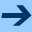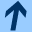NBAC format

## NBAC format

The input format of the automaton is a simple low-level format to specify dataflows equations on Booleans and numerics (integers, reals or clocks, which are reals subject to continuous evolution). An optional continuous behaviour may be specified.

#### General structure.

 ::= [] [] []

#### Types.

 ::= typedef + ::= = enum { ( (,)+ } ; ::= bool  | int  | real  | clock | | sint[]  | uint[]

Enumerated types can be defined. Labels should be different among different types, and different from variable names.

The available types for variables and expressions are resp. Booleans, integers, reals, clocks, enumerated types (referenced by their name) and bounded integers, which may be signed (sint) or unsigned (uint). The positive integer between bracket indicates the number of bits used for the encoding. Arithmetic on values of these two types follows the rule of microprocessor's arithmetic.

Clocks are special real variables which may be subject to a continuous evolution. A standard real variable does not evolve during a continuous evolution: its derivative is always equal to 0.

#### Declarations.

 ::= [] [] [] ::= state + ::= input + ::= local + ::= ( (,)+ : ;

Possible enumerated types definitions are first declared. Then variables are declared. We distinguish state variables and input variables: at each execution step, state variables take new values computed from their current values and the values of input variables. Local variables can be considered as macros or alias.

#### Definitions and transitions.

 ::= definition + ::= transition + ::= = ; ::= ' = ;

The definitions define the values of local variables with expressions (of suitable type) depending on state, input an other local variables. Dependencies between local variables should be acyclic ! (this is checked). The transitions define the values of state variables at the next execution step in function of the current values of state, input and local variables.

#### Continuous behaviour.

In case of an hybrid automaton, the continuous behaviour is specified as follows:

 ::= continuous ; ::= ,  |

The list of derivative expression should be understood as a conjunction.

#### assertion,initial and final conditions

 ::= ()* ::=  |   |  ::= assertion ; ::= initial ; ::= (invariant | final) ;

The assertion allows to constrain the values of input variables depending on the current state. It is defined by a Boolean expression on all variables, which give for each state the possible values for input variables. The initial condition defines the set of initial states by a Boolean expression on state variables, the final condition the set of invariant or final states by a Boolean expression on state variables. Invariant is the same as the negation of final.

If the assertion directive is omitted, the assertion is true.
If the initial or final directives are omitted, they are set to false.

#### Control structure.

 ::= automaton + + ::= location : ; ::= edge ( , ) : ; | edge ( , ) : , ;

A explicit control structure, defined on the previously defined dataflow system, is specified by a set of locations and edges. A location is defined by a state condition, which give the set of states represented by the location. To edges are associated a Boolean expression on state and input variables that indicate a necessary condition under which the edge can be taken by a discrete transition (first form). If a second expression is given, it indicates a necessary condition for a continuous evolution to jump from the origin location to the destination location.

The union of all state conditions of the locations indicates the considered state-space. The union of all discrete edge conditions allows to enforce the global assertion of the system.

#### Control directive.

 ::= control (, )* ;

The expressions involved in the list are supposed to be atomic conditions, that is, Boolean variables, enumerated variables, or linear inequalities. This optional directive specifies that the control structure should first be refined according to these conditions, so that in every location these variables or conditions have definite values, before resorting to refinement heuristics. These behaviour takes place after the optional initial control structure specification has been taken into account.

#### Expressions.

 ::= true  | false | | []()  | []() ::=  | / ::= |  |  | ( ) | not | | #( ) | in { } | if then else
 ::= or  | and  | xor  | eq  | xor  | => | =  | <  | <=  | >=  | > | +  | -  | * | /

Expressions are Boolean, finite type (enumerated or bounded integer), or guarded linear affine expression. Constants are either Boolean constants, integers or rational constants, or bounded integer constants, for which the type indicates how to interpret the second integer. For instance, sint(2) denotes the integer 2 encoded as a 3 bits signed integers.

The operators have the usual meaning. Arithmetic on bounded integers follows the rules of microprocessor's arithmetic. # is the exclusive operator coming from LUSTRE: it returns true if and only if at most one of its operands is true. in is mainly useful for enumerated and bounded integer types. e in {e1,e2,e3} is a shortcut for e=e1 or e=e2 or e=e3.

The priorities are: { *,/ } > { +,- } > { <, <=, =, >=,> } > { not,# } > { =, <>, xor, in } > { and } > { or } > { => } > { if then else }

#### Derivative expressions.

 ::= blocked  | anything  |  | ( ) | and | if then else
<linexpr> refers to a linear expression, without guard.
Bertrand Jeannet, February 10, 2011NBAC format